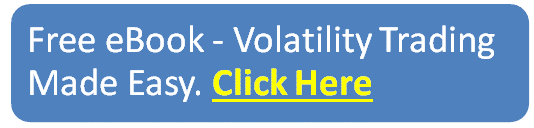blog

# Option Greeks: Everything You Need To Know

#### Options Trading 101 - The Ultimate Beginners Guide To Options

As Seen On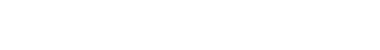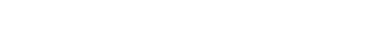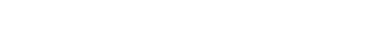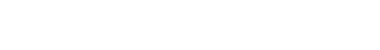by Gavin in Blog
September 10, 2021 1 commentOption greeks are incredibly important when it comes to trading options. If you think you can trade option with knowing the greeks, then you are sorely mistaken or have ben mis-informed.

The main option greeks are Delta, Gamma, Vega and Theta. There is Rho as well, but that one is less important and can pretty much be ignored.

Bookmark this page and refer back to it whenever you need, because this contains all the information you will need when it comes to option greeks.

## Contents

Option Greeks are risk assessment metrics commonly employed in the Options Market to analyze different types of risk related to such securities.

They are known as ‘The Greeks’ since each of the metrics is named after a particular Greek word.

There are five main Greeks and in this article, we will help you in understanding which kind of risk each of them measure.

If you’re a complete options beginner, you should check out my 15,000 word post on Options Trading 101.## Delta

Delta tracks the degree in which an option price changes in relation to the price of its underlying asset.

The calculation of Delta results in a range of 0 to 1 or -1 to 0 depending on the type of option (put or call) and it indicates the expected variation in the price of the option if the price of the underlying asset changes by 1%.

For example, a Delta of 0.3 indicates that if the price of the underlying asset increases by 1%, the price of the option will increase by 0.3%.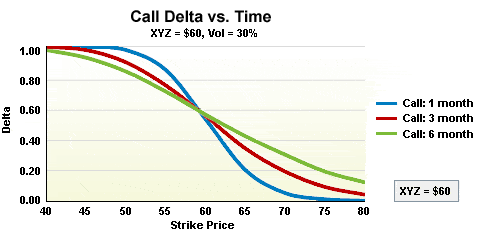RELATED ARTICLES

## Gamma

Gamma can be understood as a Delta-related calculation, as it measures the degree in which the Delta of a particular option will change if the price of the underlying asset varies by 1%.

It is, therefore, an estimation of how sensitive the Delta of the option is.

If Gamma is high, it means that the Delta of the option is highly volatile and therefore it can’t be relied on as a sound indicator of its potential behavior.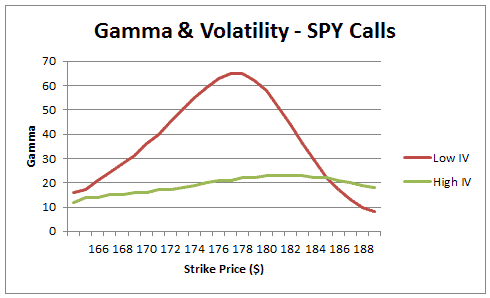RELATED ARTICLES

## Vega

Vega measures the response of an option’s price to a change in the implied volatility of its underlying security.

The implied volatility is understood as the estimated potential variation of the price of a security.

In this context, Vega indicates the potential variation in the option’s price if the implied volatility varies by 1%.

For example, a Vega of 0.7 is saying that if the implied volatility of the underlying asset changes by 1% the price of the option will vary by 0.7%.

Therefore, a security that is becoming highly volatile will increase the premium paid on the option.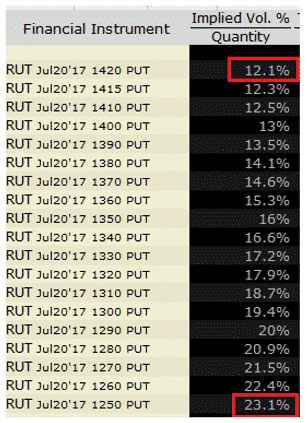RELATED ARTICLE

## Theta

Theta measures the change in the price of an option as time passes and the expiration date approaches.

Theta is commonly expressed in absolute values and an option could have a negative or positive Theta.

A negative Theta of say -0.30 indicates that each day that passes the option’s price will decrease by \$0.30.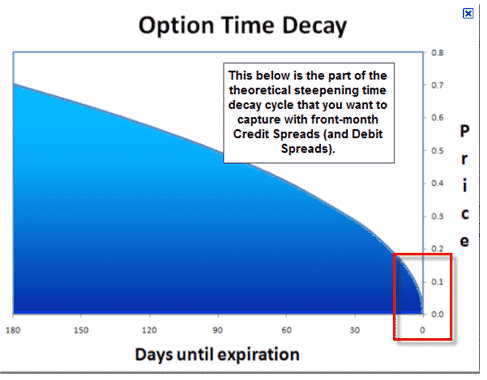RELATED ARTICLES

## Rho

Rho measures the price sensitivity of the option in relation to a change in interest rates.

The higher the Rho the more sensitive it is to potential fluctuations in interest rates.

A positive Rho means that the option will behave in the same direction as the interest rate.

If rates increase the option’s price will increase, while a negative Rho indicates that the option’s price will behave in the opposite direction of interest rates.

A Rho of 0.04 means that a 1% increase in interest rates will add \$0.04 to the price of the option.